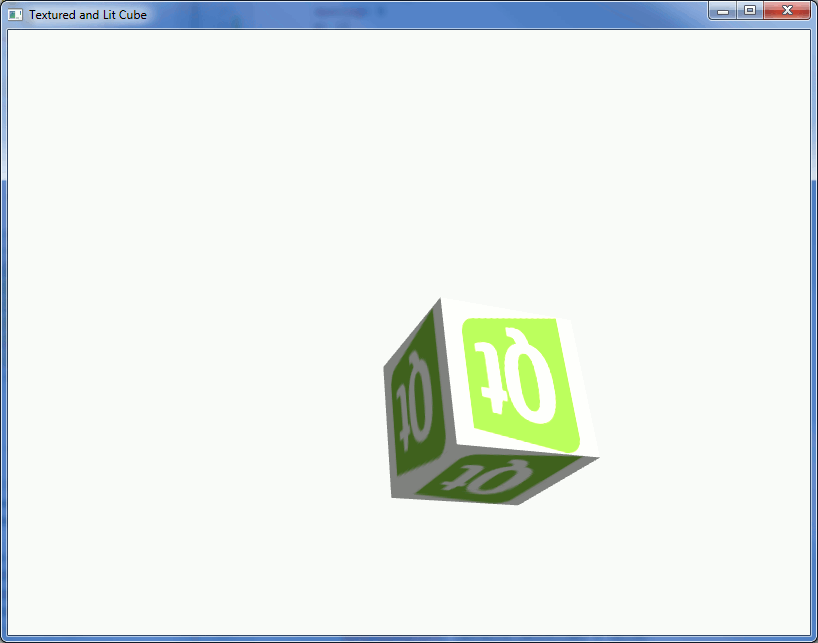# Lit and Textured Cube Example

A simple cube with texturing and lighting.

The Lit and Textured Cube example goes through the basics of using Qt Canvas 3D.## Qt Quick Implementation

### Creating Canvas3D

In main.qml, we add a Canvas3D under the root `Item`:

```Canvas3D {
id: canvas3d
anchors.fill:parent
...```

Inside it, we catch the `initializeGL` and `paintGL` signals to forward the initialization and rendering calls to the js object:

```// Emitted when one time initializations should happen
onInitializeGL: {
GLCode.initializeGL(canvas3d);
}

// Emitted each time Canvas3D is ready for a new frame
onPaintGL: {
GLCode.paintGL(canvas3d);
}```

### Importing the JavaScript File

We import the JavaScript file in the QML:

`import "textureandlight.js" as GLCode`

In the `initializeGL` function of the JavaScript, we initialize the OpenGL state. We also create the TextureImage and register handlers for image load success and fail signals. If the load succeeds, the OpenGL texture is created and filled with pixel data from the loaded image.

## JavaScript Implementation

### Matrix Library

In textureandlight.js, we first include a fast matrix library. Using this makes it a lot easier to handle 3D math operations such as matrix transformations:

`Qt.include("gl-matrix.js")`

### initializeGL Function

Let's take a closer look at the `initializeGL` function. It is called by Canvas3D once the render node is ready.

First of all, we need to get a Context3D from our Canvas3D. We want a context that supports depth buffer and antialising:

```// Get the OpenGL context object that represents the API we call
gl = canvas.getContext("canvas3d", {depth:true, antialias:true, alpha:false});```

Then we initialize the OpenGL state for the context:

```// Setup the OpenGL state
gl.enable(gl.DEPTH_TEST);
gl.depthFunc(gl.LESS);
gl.enable(gl.CULL_FACE);
gl.cullFace(gl.BACK);
gl.clearColor(0.98, 0.98, 0.98, 1.0);
gl.clearDepth(1.0);
gl.pixelStorei(gl.UNPACK_FLIP_Y_WEBGL, false);```

Next, let's take a look into shader initialization in the `initShaders` function, which we call in `initializeGL`. First we define the vertex shader:

```var vertexShader = getShader(gl,
"attribute highp vec3 aVertexNormal;    \
attribute highp vec3 aVertexPosition;  \
attribute highp vec2 aTextureCoord;    \
\
uniform highp mat4 uNormalMatrix;      \
uniform mat4 uMVMatrix;                \
uniform mat4 uPMatrix;                 \
\
varying mediump vec4 vColor;           \
varying highp vec2 vTextureCoord;      \
varying highp vec3 vLighting;          \
\
void main(void) {                      \
gl_Position = uPMatrix * uMVMatrix * vec4(aVertexPosition, 1.0); \
vTextureCoord = aTextureCoord;                                   \
highp vec3 ambientLight = vec3(0.5, 0.5, 0.5);                   \
highp vec3 directionalLightColor = vec3(0.75, 0.75, 0.75);       \
highp vec3 directionalVector = vec3(0.85, 0.8, 0.75);            \
highp vec4 transformedNormal = uNormalMatrix * vec4(aVertexNormal, 1.0); \
highp float directional = max(dot(transformedNormal.xyz, directionalVector), 0.0); \
vLighting = ambientLight + (directionalLightColor * directional); \

```var fragmentShader = getShader(gl,
"varying highp vec2 vTextureCoord;  \
varying highp vec3 vLighting;      \
\
uniform sampler2D uSampler;        \
\
void main(void) {                  \
mediump vec3 texelColor = texture2D(uSampler, vec2(vTextureCoord.s, vTextureCoord.t)).rgb; \
gl_FragColor = vec4(texelColor * vLighting, 1.0);                                      \

Then we need to create the shader program (Canvas3DProgram), attach the shaders to it, and then link and use the program:

```// Create the Canvas3DProgram for shader

}

// Take the shader program into use

And finally, look up and store the vertex attributes and uniform locations:

```// Look up where the vertex data needs to go
gl.enableVertexAttribArray(vertexPositionAttribute);
gl.enableVertexAttribArray(textureCoordAttribute);
gl.enableVertexAttribArray(vertexNormalAttribute);

// Get the uniform locations

// Setup texture sampler uniform
gl.activeTexture(gl.TEXTURE0);
gl.uniform1i(textureSamplerUniform, 0);
gl.bindTexture(gl.TEXTURE_2D, 0);```

After initializing the shader program, we set up the vertex buffer in `initBuffers` function. Let's look at the vertex index buffer creation as an example:

```var cubeVertexIndexBuffer = gl.createBuffer();
gl.bindBuffer(gl.ELEMENT_ARRAY_BUFFER, cubeVertexIndexBuffer);
gl.bufferData(gl.ELEMENT_ARRAY_BUFFER,
new Uint16Array([
0,  1,  2,      0,  2,  3,    // front
4,  5,  6,      4,  6,  7,    // back
8,  9,  10,     8,  10, 11,   // top
12, 13, 14,     12, 14, 15,   // bottom
16, 17, 18,     16, 18, 19,   // right
20, 21, 22,     20, 22, 23    // left
]),
gl.STATIC_DRAW);```

Above, first we create the buffer, then bind it and finally insert the data into it. Other buffers are all handled in a similar fashion.

As the final step in `initializeGL`, we create a texture image from TextureImageFactory, and register handlers for `imageLoaded` and `imageLoadingFailed` signals. Once the texture image is successfully loaded, we create the actual texture:

```qtLogoImage.imageLoaded.connect(function() {
// Create the Canvas3DTexture object
cubeTexture = gl.createTexture();
// Bind it
gl.bindTexture(gl.TEXTURE_2D, cubeTexture);
// Set the properties
gl.texImage2D(gl.TEXTURE_2D,    // target
0,                // level
gl.RGBA,          // internalformat
gl.RGBA,          // format
gl.UNSIGNED_BYTE, // type
qtLogoImage);     // pixels
// Set texture filtering parameters
gl.texParameteri(gl.TEXTURE_2D, gl.TEXTURE_MAG_FILTER, gl.LINEAR);
gl.texParameteri(gl.TEXTURE_2D, gl.TEXTURE_MIN_FILTER, gl.LINEAR_MIPMAP_NEAREST);
// Generate mipmap
gl.generateMipmap(gl.TEXTURE_2D);
});```

### paintGL Function

`paintGL` is called by Canvas3D whenever it is ready to receive a new frame. Let's go through the steps that are done in each render cycle.

First we check if canvas has been resized or if pixel ratio has changed, and update the projection matrix if necessary:

```var pixelRatio = canvas.devicePixelRatio;
var currentWidth = canvas.width * pixelRatio;
var currentHeight = canvas.height * pixelRatio;
if (currentWidth !== width || currentHeight !== height ) {
width = currentWidth;
height = currentHeight;
gl.viewport(0, 0, width, height);
mat4.perspective(pMatrix, degToRad(45), width / height, 0.1, 500.0);
gl.uniformMatrix4fv(pMatrixUniform, false, pMatrix);
}```

Then we clear the render area using the clear color set in `initializeGL`:

`gl.clear(gl.COLOR_BUFFER_BIT | gl.DEPTH_BUFFER_BIT);`

Next we reset the model view matrix and apply translation and rotations:

```mat4.identity(mvMatrix);
mat4.translate(mvMatrix, mvMatrix, [(canvas.yRotAnim - 120.0) / 120.0,
(canvas.xRotAnim -  60.0) / 50.0,
-10.0]);
mat4.rotate(mvMatrix, mvMatrix, degToRad(canvas.xRotAnim), [0, 1, 0]);
mat4.rotate(mvMatrix, mvMatrix, degToRad(canvas.yRotAnim), [1, 0, 0]);
mat4.rotate(mvMatrix, mvMatrix, degToRad(canvas.zRotAnim), [0, 0, 1]);
gl.uniformMatrix4fv(mvMatrixUniform, false, mvMatrix);```

As we have a lit cube, we invert and transpose the model view matrix to be used for lighting calculations:

```mat4.invert(nMatrix, mvMatrix);
mat4.transpose(nMatrix, nMatrix);
gl.uniformMatrix4fv(nUniform, false, nMatrix);```

And finally we draw the cube:

`gl.drawElements(gl.TRIANGLES, 36, gl.UNSIGNED_SHORT, 0);`

## Logging

Qt Canvas 3D uses Qt's categorized logging feature. This example enables all Qt Canvas 3D log output with the code shown below. For more on Canvas3D's logging features refer to Qt Canvas 3D Logging.

```// Uncomment to turn on all the logging categories of Canvas3D
//    QString loggingFilter = QString("qt.canvas3d.info.debug=true\n");
//    loggingFilter += QStringLiteral("qt.canvas3d.rendering.debug=true\n")
//            + QStringLiteral("qt.canvas3d.rendering.warning=true\n")
//            + QStringLiteral("qt.canvas3d.glerrors.debug=true");
//    QLoggingCategory::setFilterRules(loggingFilter);```

Files:

Images: